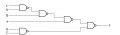# Gate Delays

Status
Not open for further replies.

#### Fatima Rashd

Joined Dec 27, 2015
4
Assume that tpd is the average of tPHL and tPLH. Find the delay from each input to the output in the circuit shown below by

(a) Finding tPHL and tPLH for each path, assuming tPHL = 0.20 ns and tPLH = 0.36 ns for each gate. From these values, find tpd for each path.

(b) Using tpd = 0.28 ns for each gate.

(c) Compare your answers from parts (a) and (b) and discuss any differences.
tPHL-C,D to F = 2 tPLH + 2tPHL = 2(0.36) + 2(0.20) = 1.12 ns
tPLH-C,D to F = 2tPHL + 2tPLH = 2(0.20) + 2(0.36) = 1.12 ns.
Please anyone explain the answer.Thank You.#### bertus

Joined Apr 5, 2008
22,217
Hello,